# Visualizing fields at points using glyphs¶

Glyphs are graphical objects that are used to represent information at points within a model. These glyphs can be coloured, scaled, and oriented according to the values of chosen fields. Glyphs might be used for something as simple as showing node locations (Figure 1), or something more complex such as showing the strain at points within a deformed mesh. cmgui has a range of glyphs available, for representing different types of point data. This document will explain how to position, scale and orient glyphs in a variety of ways.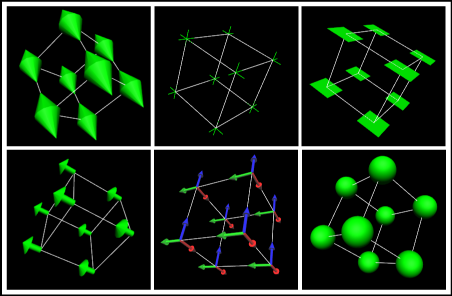Figure 1: Different glyphs placed at the nodes of a cube mesh.

## Adding glyphs to a mesh¶

Glyphs are added and edited from within the scene editor. When you select a region in the scene editor, you can create a new graphic for placing glyphs at your points of interest. Glyphs can be added at point, node points, data points, or element points. Element points are points through the interior of an element at a controllable layout and density.

## Unscaled glyphs¶

The simplest use of a glyph is to visually mark a point, with no direction or scale information. Unscaled glyphs are simply glyphs for which you have not selected an orientation/scale field. This means that they simply appear at the points you specify, at the default orientation, and with the specified the base size. The default base size is 1*1*1. If you want to run through this example, load up example a1 in cmgui and run the entire com file.

Open the scene editor and make sure cube is selected in the scene object list. You will notice that there are three items in the graphics list: lines, node_points, and surfaces. Select the node_points graphics item. You will see in the settings below that these node_points already have glyphs associated with them - in the Glyph drop-down menu, the glyphs currently used to display the nodes are point glyphs. These are the default glyph, and are by default a single white pixel. Figure 2 shows the scene editor window, with the three areas labelled.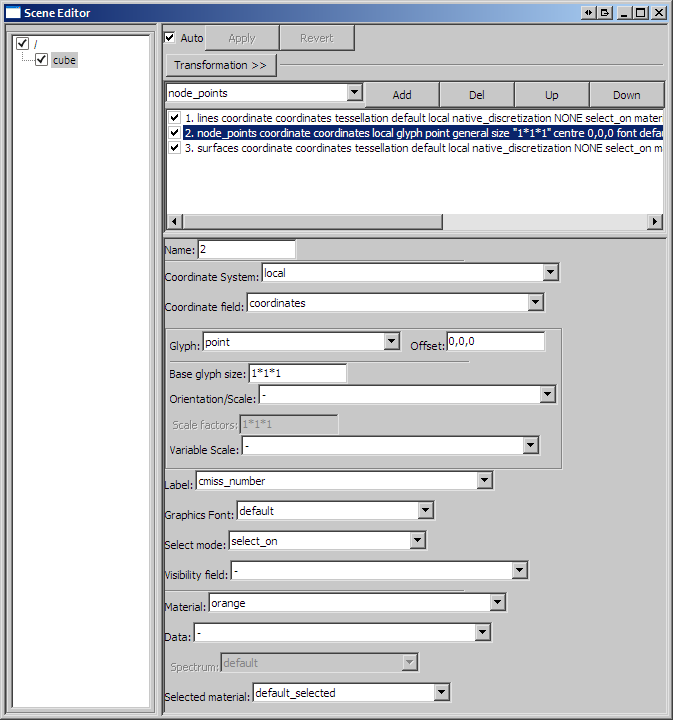Figure 2: The scene editor.

Use the drop-down menu to select another type of glyph for the node points: select sphere. In the graphics window, large orange spheres will appear at the corners (node points) of the cube. As the cube in this example is 1*1*1 and the default size of the glyphs is also 1*1*1, the glyphs will be far too large to be useful. For unscaled glyphs, you control the size by entering your desired size in the Base glyph size text-box, which will currently contain 1*1*1 (x*y*z dimensions). Change this to 0.1*0.1*0.1. If you enter a single value in the base glyph size box, it will use this value for all three dimensions. After changing the glyphs to these dimensions, you will notice that the spheres have shrunk to a more practical size (Figure 3). For unscaled glyphs, the final size is determined entirely by the Base glyph size.

Final glyph size = b1*b2*b3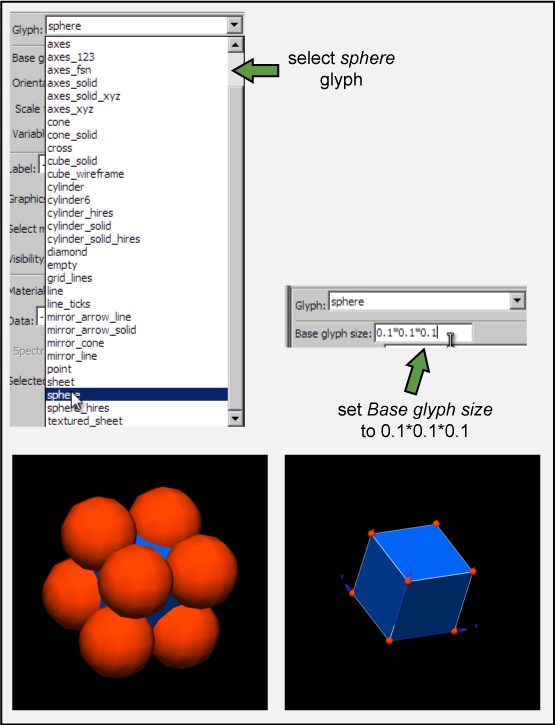Figure 3: Changing the glyph and its base size.

This is the most basic use of glyphs - to indicate points such as nodes. Other uses of glyphs allow you to represent data in addition to simple location by using the size, shape, and colour of glyphs.

## Scalar glyphs¶

Scalar glyphs can be used to represent the value of a scalar field at selected points. They have a single component orientation/scale field, which we will call S. For this case the value of S is used for all three directions of glyph scaling. The size of each glyph dimension (x, y, and z) is determined by the base size (which we will call b) plus the scale factor (which we will call c) multiplied by the orientation/scale field.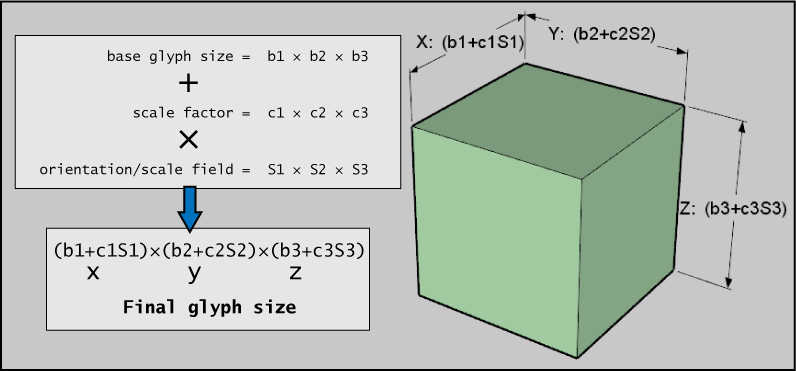Figure 4: How glyphs are scaled by a scalar orientation/scale field.

Any scale factors that are given a zero value do not add anything to the base size - in this way it is possible to scale glyphs in one, two, or three dimensions simply by using a zero value for the dimensions along which you do not wish them to scale. For example, for glyphs that only scale in the z direction, c1, c2, and c3 could be 0, 0, and 0.1 respectively. It can also be useful to set the base size to zero in order that the final glyph size is exactly proportional to the orientation/scale field.

## Vector glyphs¶

Glyphs used to represent a single vector most often use a three component orientation/scale field, but it is possible to use a two component field for two-dimensional vectors. The alignment of the glyph is determined by the alignment of the vector defined by the field; the glyph's first direction aligns to the vector, and its second and third directions are arbitrarily aligned orthogonal to this.

The glyph scaling is proportional to the magnitude of the vector, which we will call M. All three directions of the glyph are scaled by this value.

Final glyph size = (b1+c1M)*(b2+c2M)*(b3+c3M)

In most cases, b1 (base size one) is set to zero so that the final length of the glyphs is directly proportional to M; that is, their size in direction one is entirely determined by the scaling factor c1 multiplied by the magnitude M. Likewise, c2 and c3 can be set to zero so that the width and height of the glyphs are constant; in this case b2 and b3 would be set to the desired constant sizes in these directions.

## Two vector glyphs¶

These are rarely used. They have either four (2D vectors) or six (3D vectors) component orientation/scale fields. Vector 1 is defined by the first 2 or 3 components, and vector 2 by the second 2 or 3 components. The glyphs will orient their first direction along vector 1, and their second direction along vector 2. The glyph's direction 3 direction 3 is equal to the cross product of vectors 1 and 2.

The glyph scaling is proportional to the magnitude of vector 1 (M1) in direction 1, the magnitude of vector 2 (M2) in direction 2, and the cross product of vectors 1 and 2 in direction 3. For two 2D vectors, cmgui assumes a z value of 0 in order to obtain the cross product.

M1 = magnitude of vector 1 M2 = magnitude of vector 2 M3 = magnitude of cross product of vectors 1 and 2

Final glyph size = (b1+c1M1)*(b2+c2M2)*(b3+c3M3)

## Three vector glyphs¶

Three vector glyphs use a nine component orientation/scale field: vector 1 is defined by components 1,2,3, vector 2 by components 4,5,6, and vector 3 by components 7,8,9. The glyph is oriented in directions 1, 2, and 3 by the directions of vectors 1, 2, and 3 respectively. The scaling along the three directions is determined by the magnitude of the three vectors.

Final glyph size = (b1+c1M1)*(b2+c2M2)*(b3+c3M3)

### Using the fibre field¶

A special case of three vector glyphs is when you choose a fibre field for the scale/orientation field. This option automatically creates a three vector "fibre axes" field from it together with the coordinate field used by that graphic. This is equivalent to defining a field using the command gfx define field NAME fibre_axes

## Variable scale glyphs¶

Variable scale glyphs use an extra "signed scale" field to give a signed magnitude; this not only multiplies the magnitude of the orientation_scale field (so it is doubly-scaled) but its magnitude provides its "sense". A good example of this would be extension (positive) versus compression (negative) for strain. Negative values of the variable scale field reverse glyphs about their origin along their orientation. The glyph 'Repeat mode' allows glyphs to be mirrored which is useful with line, cone and arrow glyphs to help visualise principal stain and stress fields.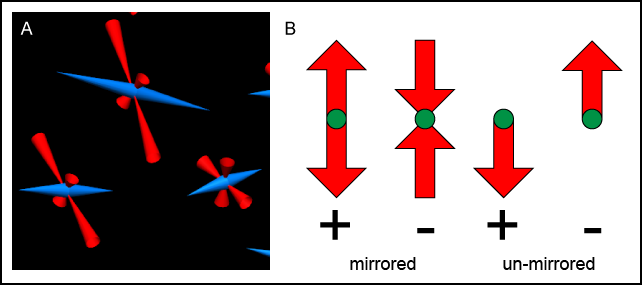Figure 5: Mirror glyphs and glyph reversal using the variable scale field. A) Mirror-cone glyphs being used in the large strain example, with the magnitude and sign of strain indicated by the length and direction of the glyphs respectively. B) How glyphs are represented with differently signed variable scale fields. Un-mirrored glyphs are not as useful for representing this information.

Variable scale glyphs need both:

• direction: orientation_scale field
• magnitude: variable_scale field

The variable scale field is an extra scaling factor in addition to the magnitude of the vector. For the final glyph size equation I will call the variable scale field "lambda" - this is because the variable scale field is often the eigenvalue of an eignevector calculated from deformations. Run through the large_strain example (a/large_strain) to see this in action.

Final glyph size = (b1+c1M*lambda1)*(b2+c2M*lambda2)*(b3+c3M*lambda3)

It is most common to use a variable scale field with single vector glyphs, such as in the large strain example.

All glyphs have a default origin; this is the point which is positioned at the chosen point within the graphical representation. This can be edited by entering values in the offset value box in the settings editor. This appears next to the glyph drop-down menu.

By default, glyphs have a 0,0,0 coordinate point (origin) that is logically positioned according to the purpose of the glyph. For directional glyphs, the "long axis" is always the x axis. Spheres, cubes and cylinders have their origin positioned in the spatial centre of a bounding unit cube. Directional glyphs such as arrows have their origin at the base of the arrow, and axis glyphs have their origin at the intersection of the axes.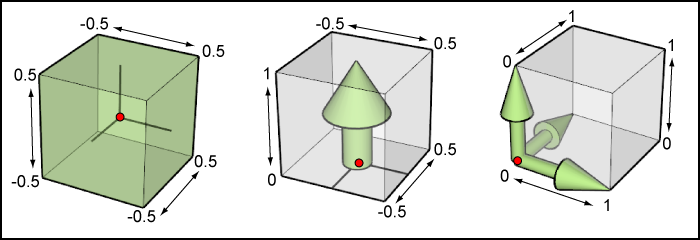Figure 6: Origins of various glyph types within their bounding cubes. Origin of each glyph family is indicated by a red dot.

Using the offset value box, you can adjust the position of your selected glyph.

### Using custom glyphs¶

It is possible in cmgui to create your own glyphs from obj model files. An example of this in action is the biplane example, where a model of a biplane is used to create a custom glyph.